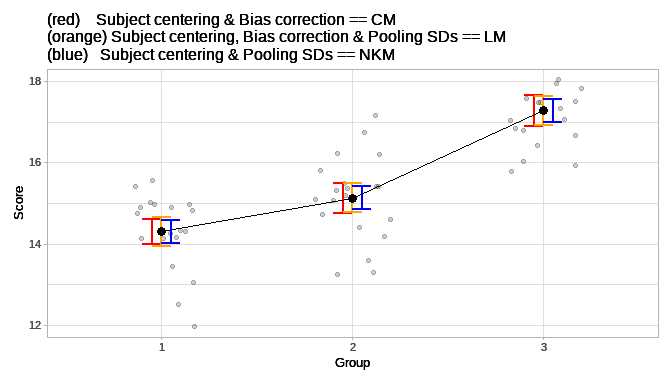# (advanced) Alternate ways to decorrelate repeated measures from transformations

The methods CM (Cousineau, 2005; Morey, 2008) and LM (Loftus & Masson, 1994) can be unified when the transformations they required are considered. In the Cousineau-Morey method, the raw data must be subject-centered and bias-corrected.

Subject centering is obtained from

$Y_{ij} = X_{ij} - \bar{X}_{i\cdot} + \bar{\bar{X}}$

in which $$i = 1..n$$ and $$j=1..C$$ where $$n$$ is the number of participants and $$C$$ is the number of repeated measures (sometimes noted with $$J$$).

Bias-correction is obtained from

$Z_{ij} = \sqrt{\frac{C}{C-1}} \left( Y_{ij} - \bar{Y}_{\cdot{}j} \right) + \bar{Y}_{\cdot{}j}$

These two operations can be performed with two matrix transformations. In comparison, the LM method requires one additional step, that is, pooling standard deviation, also achievable with the following transformation.

$W_{ij} = \sqrt{\frac{S_p^2}{S_i^2}} \left( Z_{ij} - \bar{Z}_{\cdot{}j} \right) + \bar{Z}_{\cdot{}j}$

in which $$S_j^2$$ is the variance in measurement $$j$$ and $$S_p^2$$ is the pooled variance across all $$j$$ mesurements.

With this approach, we can categorize all the proposals to repeated measure precision as requiring or not certain transformations. Table 1 shows these.

Table 1. Transformations required to implement one of the repeated-measures method. Preprocessing must precede post-processing

Method preprocessing postprocessing
Stand-alone - -
Cousineau, 2005 Subject-centering - -
CM Subject-centering Bias-correction
NKM Subject-centering - pool standard deviations
LM Subject-centering Bias-correction pool standard deviations

From that point of view, we see that the Nathoo, Kilshaw and Masson NKM (Nathoo, Kilshaw, & Masson, 2018; but see Heck, 2019 ) method is missing a bias-correction transformation, which explains why these error bars are shorter. The original proposal found in Cousineau, 2005, is also missing the bias correction step, which led Morey (2008) to supplement this approach. With these four approaches, we have exhausted all the possible combinations regarding decorrelation methods based on subject-centering.

We added two arguments in superbPlot to handle this transformation approach, the first is preprocessfct and the second is postprocessfct.

Assuming a dataset dta with replicated measures stored in say columns called Score.1, Score.2 and Score.3, the command

pCM <- superbPlot(dta, WSFactors = "moment(3)",
variables = c("Score.1","Score.2","Score.3"),
preprocessfct = "subjectCenteringTransform",
postprocessfct = "biasCorrectionTransform",
plotStyle = "pointjitter",
errorbarParams = list(color="red", width= 0.1, position = position_nudge(-0.05) )
)

will reproduce the CM error bars because it decorrelates the data as per this method. With one additional transformation,

pLM <- superbPlot(dta, WSFactors = "moment(3)",
variables = c("Score.1","Score.2","Score.3"),
preprocessfct = "subjectCenteringTransform",
postprocessfct = c("biasCorrectionTransform","poolSDTransform"),
plotStyle = "line",
errorbarParams = list(color="orange", width= 0.1, position = position_nudge(-0.0) )
)

the LM method is reproduced. Finally, if the biasCorrectionTransform is omitted, we get the NKM error bars with:

pNKM <- superbPlot(dta, WSFactors = "moment(3)",
variables = c("Score.1","Score.2","Score.3"),
preprocessfct = "subjectCenteringTransform",
postprocessfct = c("poolSDTransform"),
plotStyle = "line",
errorbarParams = list(color="blue", width= 0.1, position = position_nudge(+0.05) )
)

In what follow, I justapose the three plots to see the differences:

tlbl <- paste( "(red)    Subject centering & Bias correction == CM\n",
"(orange) Subject centering, Bias correction & Pooling SDs == LM\n",
"(blue)   Subject centering & Pooling SDs == NKM", sep="")

ornate <- list(
xlab("Group"),
ylab("Score"),
labs(   title=tlbl),
coord_cartesian( ylim = c(12,18) ),
theme_light(base_size=10)
)

# the plots on top are made transparent
pCM2 <- ggplotGrob(pCM + ornate)
pLM2 <- ggplotGrob(pLM + ornate + makeTransparent() )
pNKM2 <- ggplotGrob(pNKM + ornate + makeTransparent() )

# put the grobs onto an empty ggplot
ggplot() +
annotation_custom(grob=pCM2) +
annotation_custom(grob=pLM2) +
annotation_custom(grob=pNKM2)Figure 1. Plot of the tree decorrelation methods based on subject transformation.

The method from Cousineau (2005) missing the bias-correction step is not shown as it should not be used.

## In summary

All the decorrelation methods based on transformations have (probably) been explored. An alternative approach using correlation was proposed in Cousineau (2019). All these approaches requires sphericity of the data. Other approaches are required to overcome this sphericity limitations.